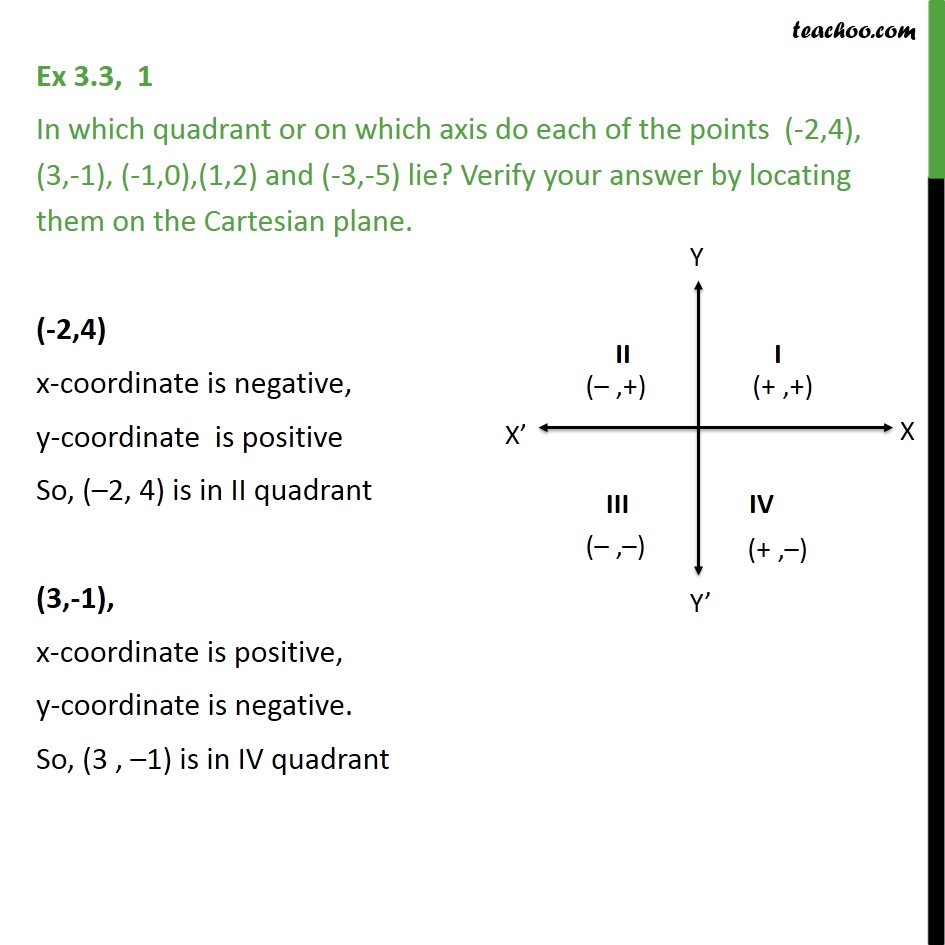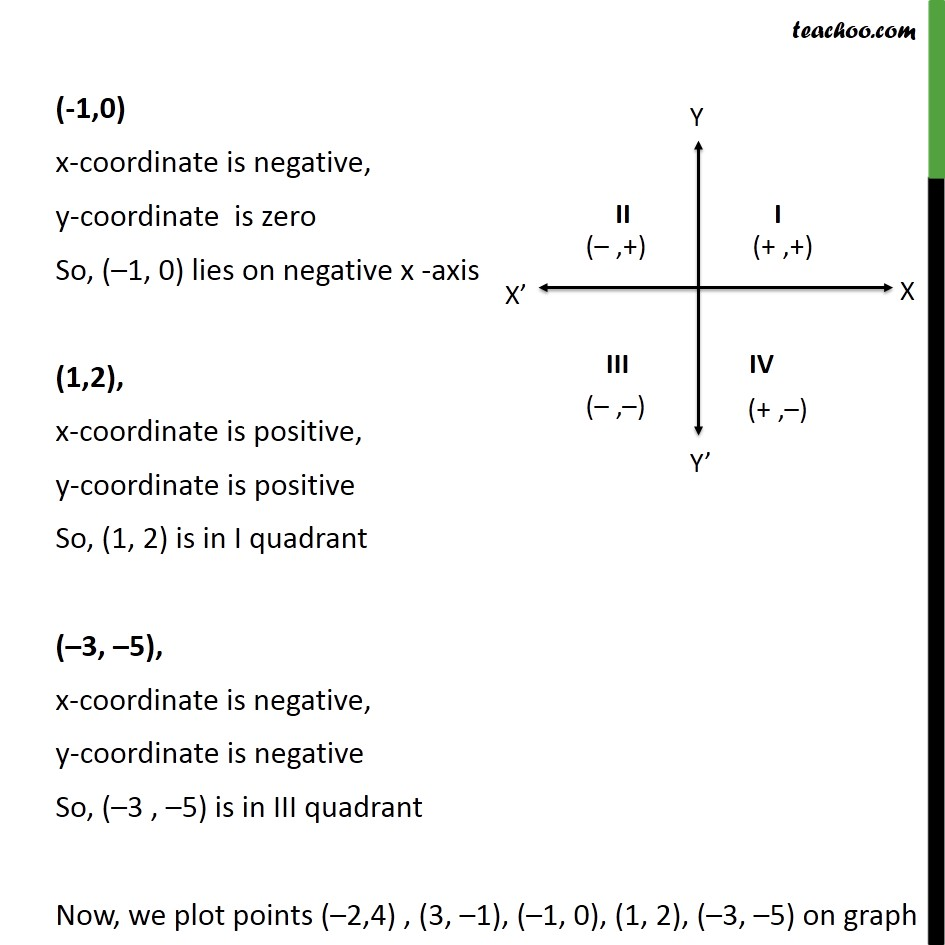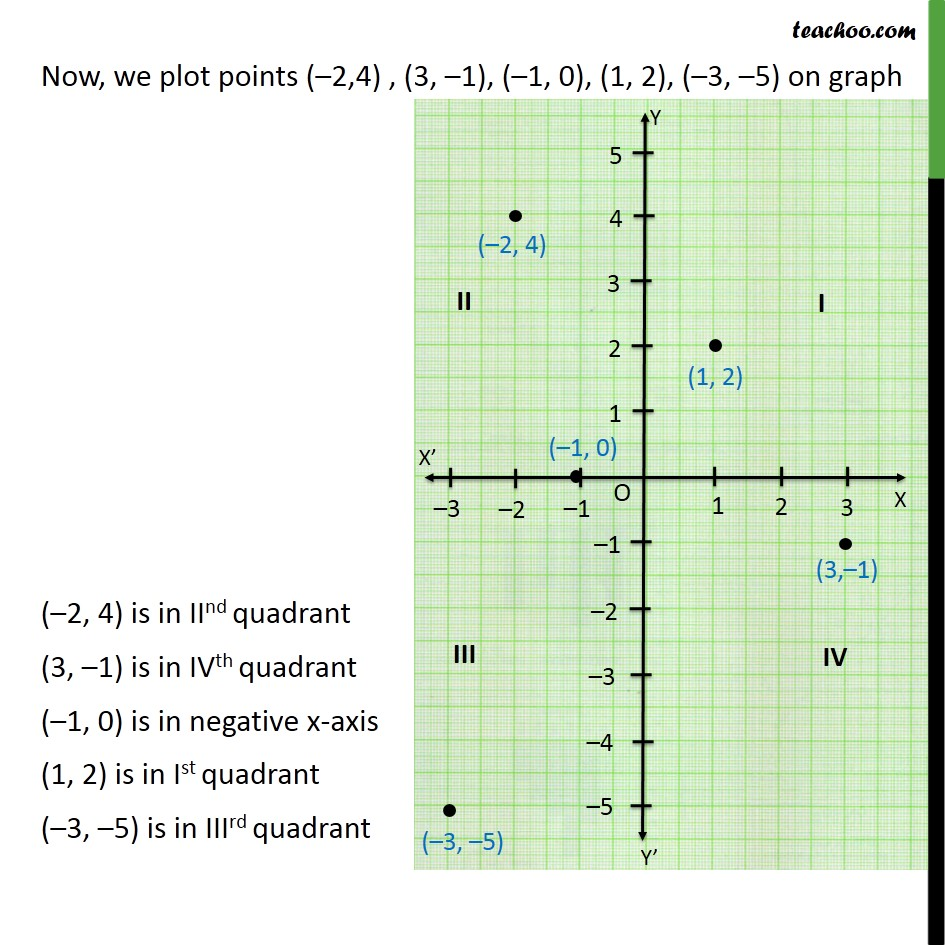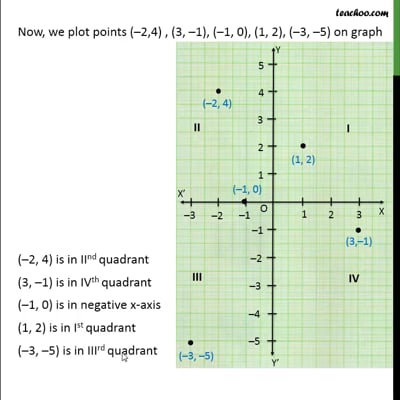Ex 3.3

Chapter 3 Class 9 Coordinate Geometry
Serial order wiseThis video is only available for Teachoo black users

Solve all your doubts with Teachoo Black (new monthly pack available now!)

### Transcript

Ex 3.3, 1 In which quadrant or on which axis do each of the points (-2,4), (3,-1), (-1,0),(1,2) and (-3,-5) lie? Verify your answer by locating them on the Cartesian plane. (-2,4) x-coordinate is negative, y-coordinate is positive So, ( 2, 4) is in II quadrant (3,-1), x-coordinate is positive, y-coordinate is negative. So, (3 , 1) is in IV quadrant (-1,0) x-coordinate is negative, y-coordinate is zero So, ( 1, 0) lies on negative x -axis (1,2), x-coordinate is positive, y-coordinate is positive So, (1, 2) is in I quadrant ( 3, 5), x-coordinate is negative, y-coordinate is negative So, ( 3 , 5) is in III quadrant Now, we plot points ( 2,4) , (3, 1), ( 1, 0), (1, 2), ( 3, 5) on graph Now, we plot points ( 2,4) , (3, 1), ( 1, 0), (1, 2), ( 3, 5) on graph ( 2, 4) is in IInd quadrant (3, 1) is in IVth quadrant ( 1, 0) is in negative x-axis (1, 2) is in Ist quadrant ( 3, 5) is in IIIrd quadrant